Courses

# RD Sharma Solutions -Ex-9.2, Triangle And Its Angles, Class 9, Maths Class 9 Notes | EduRev

## Class 9 : RD Sharma Solutions -Ex-9.2, Triangle And Its Angles, Class 9, Maths Class 9 Notes | EduRev

The document RD Sharma Solutions -Ex-9.2, Triangle And Its Angles, Class 9, Maths Class 9 Notes | EduRev is a part of the Class 9 Course RD Sharma Solutions for Class 9 Mathematics.
All you need of Class 9 at this link: Class 9

Q1) The exterior angles, obtained on producing the base of a triangle both ways are 1040  and 1360. Find all the angles of the triangle.

Solution: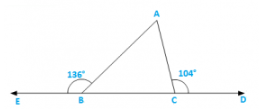∠ACD = ∠ABC + ∠BAC [Exterior angle property]
Now∠ABC = 1800 − 1360 = 440 [Linera pair]
∠ACB = 1800 − 1040 = 760 [Linera pair]
Now,InΔABC
∠A + ∠ABC + ∠ACB = 180[Sum of all angles of a triangle]
⇒ ∠A + 44+ 76= 1800
⇒ ∠A = 1800 − 44− 760
⇒ ∠A = 600

Q2) In a triangle ABC, the internal bisectors of ∠B and ∠C meet at P and the external bisectors of ∠B and ∠C meet at Q. Prove that ∠BPC + ∠BQC = 1800.

Solution: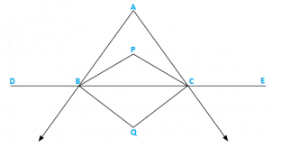Let ∠ABD = 2x and ∠ACE = 2y
∠ABC = 180− 2x [Linera pair]
∠ACB = 180− 2y [Linera pair]
∠A + ∠ABC + ∠ACB = 180[Sum of all angles of a triangle]
⇒ ∠A + 1800 − 2x + 180− 2y = 1800
⇒ − ∠A + 2x + 2y = 1800
⇒ x + y = 900 +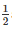∠A

Now in ΔBQC x + y + ∠BQC = 1800 [Sum of all angles of a triangle]
⇒ 90+∠A + ∠BQC = 1800
⇒ ∠BQC = 90∠A….(i)
and we know that∠BPC = 900 +∠A….(ii)
Adding (i) and (ii) we get ∠BPC + ∠BQC = 1800

Hence proved.

Q3) In figure 9.30, the sides BC, CA and AB of a triangle ABC have been produced to D, E and F respectively. If ∠ACD = 1050 and ∠EAF = 450, find all the angles of the triangle ABC.

Solution: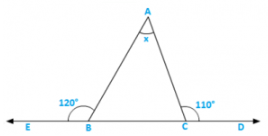∠BAC = ∠EAF = 450 [Verticallyopposite angles]
∠ABC = 1050 − 450 = 600 [Exterior angle property]
∠ACD = 1800 − 1050 = 750 [Linear pair]

Q4) Compute the value of x in each of the following figures:

(i)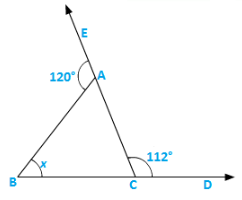Solution:

∠BAC = 1800 − 1200 = 600 [Linear pair]
∠ACB = 180− 1120 = 680 [Linear pair]
∴ x = 180− ∠BAC − ∠ACB
= 1800 − 600 − 680 = 520 [Sum of all angles of a triangle]

(ii)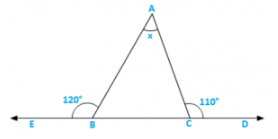Solution:

∠ABC = 1800 − 120= 600 [Linear pair]
∠ACB = 180− 1100 = 700 [Linear pair]
∴ e∠BAC = x = 1800 − ∠ABC − ∠ACB
= 1800 − 60− 700 = 500 [Sum of all angles of a triangle]

(iii)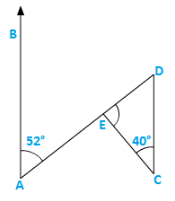Solution:

∠BAE = ∠EDC = 520 [Alternate angles]
∴∠DEC = x = 1800 − 400 − ∠EDC = 1800 − 400 − 52
= 1800 − 920 = 880 [Sum of all angles of a triangle]

(iv)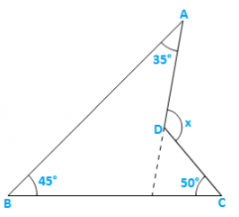Solution:

CD is produced to meet AB at E.

∠BEC = 1800 − 45− 500 = 85[Sumofall angles of a triangle]
∠AEC = 180− 85= 950 [Linear pair]
∴x = 950 + 350 = 1300 [Exterior angle property] .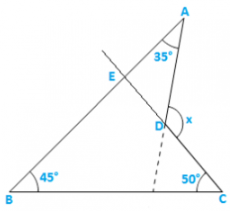Q5) In figure 9.35, AB divides ∠DAC in the ratio 1 : 3 and AB = DB. Determine the value of x.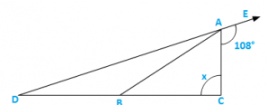Solution:

⇒ ∠BDA = ∠BAD = Z           (∵AB = DB)
Now∠BAD + ∠BAC + 1080 = 1800 [Linear pair]
⇒ Z + 3Z + 108= 1800
⇒ 4Z = 720
⇒ Z = 180
⇒ x + 180 = 1800
⇒ x = 900

Q6) ABC is a triangle. The bisector of the exterior angle at B and the bisector of ∠C intersect each other at D. Prove that ∠D =∠A.

Solution: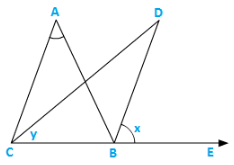Let ∠ABE = 2x and ∠ACB = 2y ∠ABC = 1800 − 2x [Linear pair]
∴∠A = 1800 − ∠ABC − ∠ACB [ angle sum property]
= 180− 1800 + 2x + 2y = 2(x − y)…..(i)
Now,∠D = 1800 − ∠DBC − ∠DCB
⇒ ∠D = 1800 − (x + 1800 − 2x) − y
⇒ ∠D = 1800 − x − 1800 + 2x − y = (x − y) =∠A…..from(i)
Hence,∠D =∠A.

Q7) In figure 9.36, AC⊥CE and ∠A:∠B:∠C = 3:2:1,find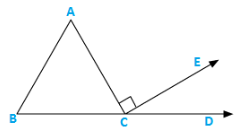Solution:

∠A:∠B:∠C = 3:2:1
Let the angles be 3x,2x and x
⇒ 3x + 2x + x = 1800 [ angle sum property]
⇒ 6x = 1800
⇒ x = 30= ∠ACB
∴∠ECD = 180− ∠ACB − 90[Linear pair]
= 1800 − 300 − 900 = 600
∴∠ECD = 600

Q8) In figure 9.37, AM⊥BC and AN is the bisector of ∠A. If ∠B = 650 and ∠C = 330,find ∠ MAN..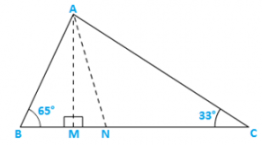Solution:

Let∠BAN = ∠NAC = x [∵AN bisects ∠A]
∴∠ANM = x + 330 [Exterior angle property]
In ΔAMB ∠BAM = 90− 65= 250 [Exterior angle property]
∴∠MAN = ∠BAN − ∠BAM = (x − 25)0
Now in ΔMAN,(x − 25)+ (x + 33)+ 900 = 180[ angle sum property]
⇒ 2x + 80 = 900
⇒ 2x = 820
⇒ x = 410
∴MAN = x − 250 = 410 − 250 = 160

Solution: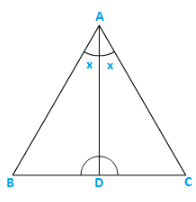∵∠C>∠B                               [Given]

⇒ ∠C + x>∠B + x                 [Adding x on both sides]

Hence proved.

Q10) In triangle ABC, BD⊥AC and CE⊥AB. If BD and CE intersect at O, prove that ∠BOC = 1800 − ∠A..

Solution: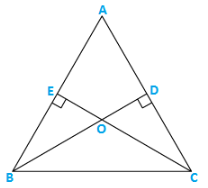∠A + ∠AEO + ∠EOD + ∠ADO = 3600
⇒ ∠A + 900 + 90+ ∠EOD = 3600
⇒ ∠A + ∠BOC = 1800 [∵∠EOD = ∠BOC  vertically opposite angles]
⇒ ∠BOC = 1800 − ∠A

Q11) In figure 9.38, AE bisects ∠CAD and ∠B = ∠C. Prove that AE∥BC.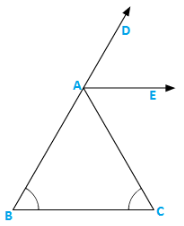Solution:

Let∠B = ∠C = x
Then,∠CAD = ∠B + ∠C = 2x (exterior angle)∠CAD = x
⇒ ∠EAC = x
⇒ ∠EAC = ∠C

These are alternate interior angles for the lines AE and BC

∴AE∥BC

Q12) In figure 9.39, AB∥DE.Find∠ACD.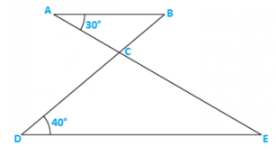Solution:

Since AB∥DE

∴∠ABC = ∠CDE = 400 [Alternate angles]
∴∠ACB = 1800 − ∠ABC − ∠BAC
= 1800 − 400 − 30= 1100
∴∠ACD = 1800 − 1100 [Linear pair] = 700

Q13) . Which of the following statements are true (T) and which are false (F) :

(i) Sum of the three angles of a triangle is 180°.

Solution: (i) T

(ii) A triangle can have two right angles.

Solution: (ii) F

(iii) All the angles of a triangle can be less than 60°.

Solution: (iii) F

(iv) All the angles of a triangle can be greater than 60°.

Solution: (iv) F

(v) All the angles of a triangle can be equal to 60°.

Solution: (v) T

(vi) A triangle can have two obtuse angles.

Solution: (vi) F

(vii) A triangle can have at most one obtuse angles.

Solution: (vii) T

(viii) If one angle of a triangle is obtuse, then it cannot be a right angled triangle.

Solution: (viii) T

(ix) An exterior angle of a triangle is less than either of its interior opposite angles.

Solution: (ix) F

(x) An exterior angle of a triangle is equal to the sum of the two interior opposite angles.

Solution: (x) T

(xi) An exterior angle of a triangle is greater than the opposite interior angles.

Solution: (xi) T

Q14) Fill in the blanks to make the following statements true:

(i) Sum of the angles of a triangle is _______ .

(ii) An exterior angle of a triangle is equal to the two ________ opposite angles.

(iii) An exterior angle of a triangle is always ________ than either of the interior opposite angles.

(iv) A triangle cannot have more than _______ right angles.

(v) A triangles cannot have more than _______ obtuse angles.

Solution:

(i) 1800

(ii) Interior

(iii) Greater

(iv) One

(v) One

Offer running on EduRev: Apply code STAYHOME200 to get INR 200 off on our premium plan EduRev Infinity!

91 docs

,

,

,

,

,

,

,

,

,

,

,

,

,

,

,

,

,

,

,

,

,

,

,

,

,

,

,

,

,

,

;